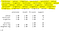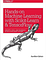# Classify Iris Species Using Python & Logistic Regression

Logistic Regression Python Program

In this article I will show you how to write a simple logistic regression program to classify an iris species as either ( virginica, setosa, or versicolor) based off of the pedal length, pedal height, sepal length, and sepal height using a machine learning algorithm called Logistic Regression.

Logistic regression is a model that uses a logistic function to model a dependent variable. Like all regression analyses, the logistic regression is a predictive analysis. Logistic regression is used to describe data and to explain the relationship between one dependent variable and one or more nominal, ordinal, interval or ratio-level independent variables.

If you prefer not to read this article and would like a video representation of it, you can check out the YouTube video below. It goes through everything in this article with a little more detail, and will help make it easy for you to start programming your own Machine Learning model in Python. Or you can use both (this article and video) as supplementary materials for learning about Machine Learning !

# Start Programming:

I will start by stating what I want this program to do. I want this program to predict/classify the iris species as either ( virginica, setosa, or versicolor) based off of the pedal length, pedal height, sepal length, and sepal height

First I will import the dependencies, that will make this program a little easier to write. I’m importing the machine learning library sklearn, seaborn, and matplotlib.

`# Import the dependenciesimport matplotlib.pyplot as pltimport seaborn as snsfrom sklearn.linear_model import LogisticRegressionfrom sklearn.metrics import classification_reportfrom sklearn.metrics import accuracy_scorefrom sklearn.model_selection import train_test_split`

Next I will load the data set from the seaborn library, store it into a variable called data, and print the first 5 rows of data.

`#Load the data setdata = sns.load_dataset("iris")data.head()`

Start preparing the training data set by storing all of the independent variables/columns/features into a variable called ‘X’, and store the independent variable/target into a variable called ‘y’.

`#Prepare the training set# X = feature values, all the columns except the last columnX = data.iloc[:, :-1]# y = target values, last column of the data framey = data.iloc[:, -1]`

Plot the relation of each feature / column with each species. I will use a scatter plot to show this relation. The sepal length will be blue, sepal width will be green, petal length will be red and petal width will be black.

`# Plot the relation of each feature with each speciesplt.xlabel('Features')plt.ylabel('Species')pltX = data.loc[:, 'sepal_length']pltY = data.loc[:,'species']plt.scatter(pltX, pltY, color='blue', label='sepal_length')pltX = data.loc[:, 'sepal_width']pltY = data.loc[:,'species']plt.scatter(pltX, pltY, color='green', label='sepal_width')pltX = data.loc[:, 'petal_length']pltY = data.loc[:,'species']plt.scatter(pltX, pltY, color='red', label='petal_length')pltX = data.loc[:, 'petal_width']pltY = data.loc[:,'species']plt.scatter(pltX, pltY, color='black', label='petal_width')plt.legend(loc=4, prop={'size':8})plt.show()`

Split the data into 80% training and 20 % testing by using the method train_test_split() from the sklearn.model_selection library, and store the data into x_train, x_test, y_train, and y_test.

`#Split the data into 80% training and 20% testingx_train, x_test, y_train, y_test = train_test_split(X, y, test_size=0.2, random_state=42)`

Create and train the Logistic Regression model !

`#Train the modelmodel = LogisticRegression()model.fit(x_train, y_train) #Training the model`

Now that the model is trained, I will print the predictions and get a few metrics from the model based off of the testing data set. Based off of the metrics, it looks like the model correctly classified every species.

`#Test the modelpredictions = model.predict(x_test)print(predictions)# printing predictionsprint()# Printing new line#Check precision, recall, f1-scoreprint( classification_report(y_test, predictions) )print( accuracy_score(y_test, predictions))`Highlighted In Yellow Is The Models Prediction. Below Are The Metrics.

That is it, you are done creating your Logistic Regression program to classify iris species ! Again if you want, you can watch and listen to me explain all of the code on my YouTube video.

If you are interested in reading more on machine learning to immediately get started with problems and examples then I strongly recommend you check out Hands-On Machine Learning with Scikit-Learn and TensorFlow: Concepts, Tools, and Techniques to Build Intelligent Systems. It is a great book for helping beginners learn how to write machine learning programs, and understanding machine learning concepts.Hands-On Machine Learning with Scikit-Learn and TensorFlow: Concepts, Tools, and Techniques to Build Intelligent Systems